Inner

Inner is a generalization of Dot in that other functions can be used in place of Times and Plus. In the next two cells we see Inner[Times, list1, list2, Plus] is equivalent to  Dot[list1, list2] .  Before demonstrating Dot I clear the values from any symbols.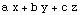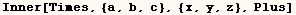The next cell shows a generic application of Inner.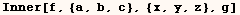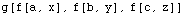In the next cell we see that when Inner is given only three arguments Plus is used in place of a fourth argument.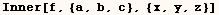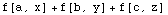In the next cell we see Inner can handle things other than lists. Inner only requires that the objects have the same Head and compatible dimensions.Inner product with Matrices and Tensors

In the section on Dot we saw that Dot can be used on lists, matrices and tensors. Inner does the same thing with Lists, matrices and tensors except other functions can be used in place of Times and Plus.

In the next cell (A) and (B) are matrices and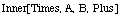is the same as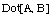.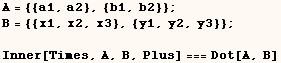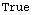In the next cell we evaluate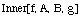and we get somthing like matrix multiplication except (f) is used in place of multiplication, and (g) is used in place of addition.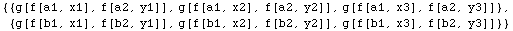I don't give examples of using Inner on tensors, but I think you will find it's very much like the Dot product of tensors.

Giving Inner a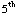argument

In the next example Inner is given (1) as a fifth argument and, (at least in this example) Inner[Times,A,B,Plus,1] is equivalent to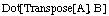.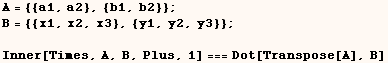The next example shows that (at least in this example) the default value of the fifth argument is 2.Created by Mathematica  (May 16, 2004)

Back to Ted’s Tricks index page